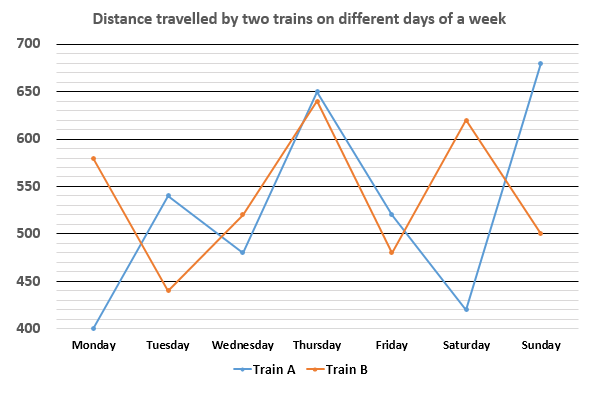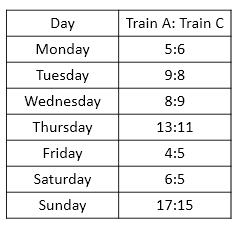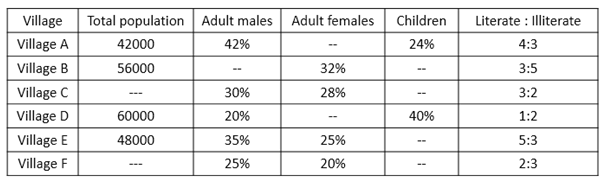# SBI Clerk/ IDBI and South Indian Bank Mains Quantitative Aptitude Questions 2019 (Day-03)

Dear Aspirants, Our IBPS Guide team is providing a new series of Quantitative Aptitude Questions for SBI Clerk /IDBI and South Indian Bank Mains 2019 so the aspirants can practice it on a daily basis. These questions are framed by our skilled experts after understanding your needs thoroughly. Aspirants can practice these new series questions daily to familiarize with the exact exam pattern and make your preparation effective.

Check here for IBPS RRB Clerk Prelims Mock Test 2019

Check here for EPFO Assistant Mock Test 2019

Check here for LIC ADO Mock Test 2019

[WpProQuiz 6711]

Directions (1 – 5): Study the following information carefully and answer the questions given below:

The following line graph shows the distance (in Km) travel by two trains on different days of a week.The following table shows the ratio of the distance travelled by train A and train C on different days of the week.1) Sum of the distances travelled by train C on Sunday and Monday together is what percent of the sum of the distances travelled by train B on the same days?

a) 50%

b) 68%

c) 75%

d) 100%

e) None of these

2) If the speed of train B on Wednesday is 39 Km/h and speed of train C on Thursday is 33 Km/h, find the respective ratio of the time taken by them to cover respective distances.

a) 2: 3

b) 3: 4

c) 4: 5

d) 3: 5

e) None of these

3) Find the difference between the distances travelled by train A on Monday, Tuesday and Wednesday together and distance travelled by train B on Thursday, Friday and Saturday together.

a) 320 Km

b) 410 Km

c) 530 Km

d) 380 Km

e) None of these

4) Time taken by train B to travel the distance on Tuesday is 11 hours and time taken by train A to travel the distance on Saturday is 10 hours. Speed of train B on Tuesday is what percent of the speed of train A on Saturday?

a) 95.23%

b) 66.67%

c) 86.67%

d) 83.33%

e) 93.33%

5) Find the sum of the distances travelled by train C throughout the week.

a) 4560 Km

b) 3650 Km

c) 4220 Km

d) 3820 Km

e) None of these

Directions (6 – 10): Study the following information carefully and answer the questions given below:

The following table represents Population of six different villages, percentage of adult males, adult females and children and ratio of literates and illiterates. Some data in the following table are missing.6) Adult female population of village A is what percent of the illiterate population of that village?

a) 91.11%

b) 79.33%

c) 86.67%

d) 53.33%

e) None of these

7) If number of literate population of village C is 30000, find the difference between number of children in village C and number of adult females in village D.

a) 1000

b) 2000

c) 3000

d) 1500

e) None of these

8) Ratio of number of adult males and number of children in village B is 10:7 respectively. Find the respective ratio of number of adult males and number of illiterates in village B.

a) 107: 175

b) 109: 113

c) 111: 139

d) 112: 175

e) None of these

9) Number of adult females in village E is what percent more/less than the number of children in village F?

a) 54.54% less

b) 45.45% less

c) 54.54% more

d) 45.45% more

e) None of these

10) Population of village C and village F is 50000 and 40000 respectively. Find the total number of adult females in all the villages.

a) 84200

b) 88400

c) 90200

d) 78600

e) None of these

Direction (1 – 5) :

Distance travelled by train C on Sunday = 15/17 x 680 = 600 Km

Distance travelled by train C on Monday = 6/5 x 400 = 480 Km

Sum of the distances travelled by train C on Sunday and Monday together

= 600 + 480 = 1080 Km

Sum of the distances travelled by train B on Sunday and Monday together

= 500 + 580 = 1080 Km

Required percentage = 1080/1080 x 100 = 100%

Time taken by train B on Wednesday to cover the distance = 520/39

= 40/3 hours

Distance travelled by train C on Thursday = 11/13 x 650 = 550 Km

Time taken by train C on Thursday to cover the distance = 550/33 = 50/3 hours

Required ratio = 40/3: 50/3 = 4: 5

Distances travelled by train A on Monday, Tuesday and Wednesday together

= 400 + 540 + 480

= 1420 Km

Distance travelled by train B on Thursday, Friday and Saturday together

= 640 + 480 + 620

= 1740 Km

Required difference = 1740 – 1420 = 320 Km

We know that

Speed = Distance/Time

Speed of train B on Tuesday = 440/11 = 40 Km/h

Speed of train A on Saturday = 420/10 = 42 Km/h

Required percentage = 40/42 x 100 = 95.23%

Distance travelled by train C on Monday = 6/5 x 400 = 480 Km

Distance travelled by train C on Tuesday = 8/9 x 540 = 480 Km

Distance travelled by train C on Wednesday = 9/8 x 480 = 540 Km

Distance travelled by train C on Thursday = 11/13 x 650 = 550 Km

Distance travelled by train C on Friday = 5/4 x 520 = 650 Km

Distance travelled by train C on Saturday = 5/6 x 420 = 350 Km

Distance travelled by train C on Sunday = 15/17 x 680 = 600 Km

Required sum = 480 + 480 + 540 + 550 + 650 + 350 + 600 = 3650 Km

Direction (6-10) :

Adult female population of village A = (100 – 42 – 24)/100 x 42000

= 34/100 x 42000

= 14280

Illiterate population of village A = 3/7 x 42000

= 18000

Required percentage = 14280/18000 x 100

= 79.33%

Total population of village C = 5/3 x 30000 = 50000

Number of children in village C = (100 – 30 – 28)/100 x 50000

= 42/100 x 50000

= 21000

Number of adult females in village D = (100 – 20 – 40)/100 x 60000

= 40/100 x 60000

= 24000

Required difference = 24000 – 21000 = 3000

Number of adult males in village B = 10/17 x (100 – 32)/100 x 56000

= 10/17 x 68/100 x 56000

= 22400

Number of illiterates in village B = 5/8 x 56000

= 35000

Required ratio = 22400: 35000

= 112: 175

Number of adult females in village E = 25/100 x 48000 = 12000

Number of children in village F = (100 – 25 – 20)/100 x 40000

= 55/100 x 40000

= 22000

Required percentage = (22000 – 12000)/22000 x 100

= 10000/22000 x 100

= 45.45% less

Number of adult females in village A = (100 – 42 – 24)/100 x 42000

= 34/100 x 42000

= 14280

Number of adult females in village B = 32/100 x 56000 = 17920

Number of adult females in village C = 28/100 x 50000 = 14000

Number of adult females in village D = (100 – 20 – 40)/100 x 60000

= 40/100 x 60000

= 24000

Number of adult females in village E = 25/100 x 48000 = 12000

Number of adult females in village F = 20/100 x 40000 = 8000

Required sum = 14280 + 17920 + 14000 + 24000 + 12000 + 8000 = 90200